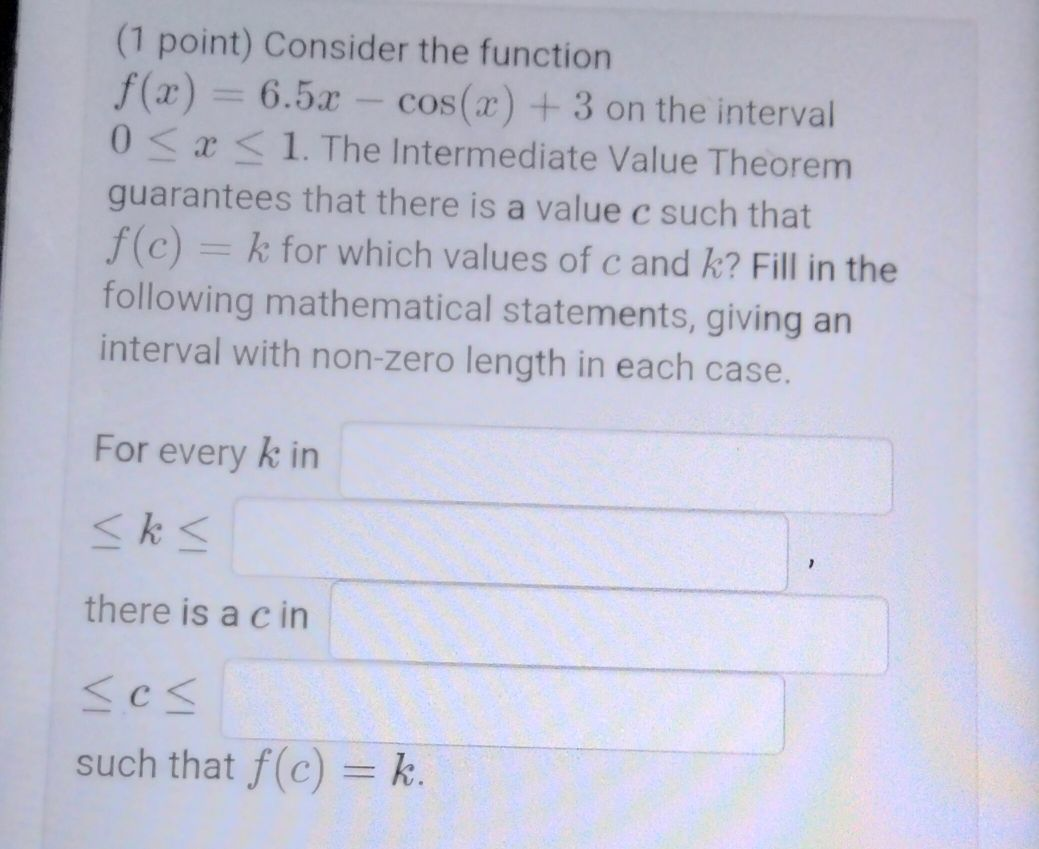### ¿Todavía tienes preguntas de matemáticas?

Pregunte a nuestros tutores expertos
Algebra
Pregunta(1 point) Consider the function $$f ( x ) = 6.5 x - \cos ( x ) + 3$$ on the interval

$$0 \leq x \leq 1$$ . The Intermediate Value Theorem guarantees that there is a value $$c$$ such that

$$f ( c ) = k$$ for which values of $$c$$ and $$k$$ ? Fill in the following mathematical statements, giving an interval with non-zero length in each case.

For every $$k$$ in

$$\leq k \leq$$

there is a $$c$$ in

$$\leq c \leq$$

such that $$f ( c ) = k$$